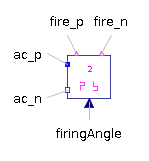Rotor Saliency Air Gap - MapleSim Help

QuasiStatic Rotor Saliency Air Gap

Air gap model with rotor saliencyDescription The QuasiStatic Rotor Saliency Air Gap component can be used for machines with uniform air gaps and for machines with rotor saliencies. The air gap model is not symmetrical towards stator and rotor because the saliency always refers to the rotor. The saliency of the air gap is represented by a main field inductance in the d- and q-axis. For the mechanical interaction of the air gap model with the stator and the rotor it is equipped with two rotational connectors.  The torques acting on both connectors have the same absolute values but different signs. The stator and the rotor reference angles, ${\mathrm{\gamma }}_{s}$ and ${\mathrm{\gamma }}_{r}$ are related by ${\mathrm{\gamma }}_{s}=\mathrm{\gamma }+{\mathrm{\gamma }}_{r}$, where $\mathrm{\gamma }$ is the electrical angle between stator and rotor. The air gap model has two magnetic stator and two magnetic rotor ports. The magnetic potential difference and the magnetic flux of the stator and rotor are equal complex quantities, respectively, but the reference angles are different. The d- and q-axis components with respect to the rotor fixed reference frame (superscript r) are determined from the stator (superscript s) and rotor (superscript r) reference quantities, byThe d- and q-axis magnetic potential difference components and flux components are related to the flux byConnections

 Name Description Modelica ID ${\mathrm{port}}_{\mathrm{sp}}$ Positive complex magnetic stator port port_sp ${\mathrm{port}}_{\mathrm{sn}}$ Negative complex magnetic stator port port_sn ${\mathrm{port}}_{\mathrm{rp}}$ Positive complex magnetic rotor port port_rp ${\mathrm{port}}_{\mathrm{rn}}$ Negative complex magnetic rotor port port_rn ${\mathrm{flange}}_{a}$ Flange of the rotor flange_a $\mathrm{support}$ Support at which the reaction torque is acting supportParameters

 Name Default Units Description Modelica ID $p$ Number of pole pairs p ${L}_{{0}_{d}}$ $H$ Salient inductance, direct-axis component L0d ${L}_{{0}_{q}}$ $H$ Salient inductance, quadrature-axis component L0qModelica Standard Library The component described in this topic is from the Modelica Standard Library. To view the original documentation, which includes author and copyright information, click here.